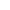Top | Introduction | Members | Activities | Call for Paper | Link |Japanese## "A study on Efficient Elliptic Curve Exponentiation"

 In the elliptic curve cryptosystem, we mainly calculate the scalar multiplication. Since only the value of x-coordinates is used at some elliptic curve cryptosystem algorithm (ECDH, ECDSA-S, etc.), the algorithm which calculate scalar multiplication at high speed without using y-coordinates is proposed. In Montgomery form elliptic curve, there is the operation method using Lucas chain as a technique equivalent to addition chain. In this research, some improvements are added to scalar multiplication algorithm with Lucas chain and the effect of each improvement in numerical experiments is shown. We proposed more efficient scalar multiplication algorithm which combined those improvements. [ back ]